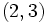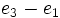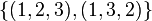# Standard representation of symmetric group:S3

(diff) ← Older revision | Latest revision (diff) | Newer revision → (diff)
This article describes a particular irreducible linear representation for the following group: symmetric group:S3. The representation is unique up to equivalence of linear representations and is irreducible, at least over its original field of definition in characteristic zero. The representation may also be definable over other characteristics by reducing the matrices modulo that characteristic, though it may behave somewhat differently in these characteristics.
For more on the linear representation theory of the group, see linear representation theory of symmetric group:S3.

This article discusses a two-dimensional faithful irreducible representation of symmetric group:S3, called the standard representation since it belongs to the family of standard representations of symmetric groups.

## Summary

Item Value
Degree of representation 2
Schur index 1 in all characteristics
Kernel of representation trivial subgroup, i.e., it is a faithful linear representation in all characteristics.
Quotient on which it descends to a faithful linear representation symmetric group:S3
Set of character values$\{ 2,0,-1 \}$ (interpreted/reduced modulo the ring or field)
Characteristic zero: Ring generated:$\mathbb{Z}$ -- ring of integers, Ideal within ring generated: whole ring, Field generated:$\mathbb{Q}$ -- field of rational numbers
Rings of realization Realized over any unital ring, by composing the representation over$\mathbb{Z}$ with the map induced by the natural homomorphism from$\mathbb{Z}$ to the ring.
Minimal ring of realization (characteristic zero)$\mathbb{Z}$ -- ring of integers
Minimal ring of realization in characteristic$p^k$ The ring of integers mod$p^k$,$\mathbb{Z}/p^k\mathbb{Z}$
Minimal field of realization Prime field in all cases.
In characteristic zero,$\mathbb{Q}$; in characteristic$p$, the field$\mathbb{F}_p$
Size of equivalence class under automorphisms 1, because the group is a complete group, so there are no outer automorphism classes to worry about. (see symmetric groups are complete).
Size of equivalence class under Galois automorphisms 1, because the representation is realized over$\mathbb{Z}$ in characteristic zero and more generally is realized over the prime subfield in any characteristic. See symmetric groups are rational-representation)
Bad characteristics 3: in characteristic 3, the representation can be defined and is indecomposable but not irreducible (more below).

## Representation table

The representation can be defined in many equivalent ways. Note that the first two description columns show that the representation can be realized over$\mathbb{Z}$. In particular, this allows us to define it over any field and more generally over any unital ring by composing with the homomorphism from$\mathbb{Z}$ to that ring. In characteristic 3, however, the representation is not irreducible (as discussed later).

Element Matrix for standard representation with basis$e_1 - e_2$,$e_2 - e_3$ Matrix for standard representation viewed as quotient with basis$\overline{e_1}, \overline{e_2}$ Matrix for real representation as dihedral group Matrix for complex representation as dihedral group Characteristic polynomial Minimal polynomial Trace, character value Determinant
Identity element$\begin{pmatrix} 1 & 0 \\ 0 & 1 \\\end{pmatrix}$$\begin{pmatrix} 1 & 0 \\ 0 & 1 \\\end{pmatrix}$$\begin{pmatrix} 1 & 0 \\ 0 & 1 \\\end{pmatrix}$$\begin{pmatrix} 1 & 0 \\ 0 & 1 \\\end{pmatrix}$$(t - 1)^2$$t - 1$ 2 1$(1,2,3)$$\begin{pmatrix} 0 & -1 \\ 1 & -1 \\\end{pmatrix}$$\begin{pmatrix} 0 & -1 \\ 1 & -1 \\\end{pmatrix}$$\begin{pmatrix} -1/2 & -\sqrt{3}/2 \\ \sqrt{3}/2 & -1/2\end{pmatrix}$$\begin{pmatrix} e^{2\pi i/3} & 0 \\ 0 & e^{-2\pi i/3}\end{pmatrix}$$t^2 + t + 1$$t^2 + t + 1$ -1 1$(1,3,2)$$\begin{pmatrix} -1 & 1 \\ -1 & 0 \\\end{pmatrix}$$\begin{pmatrix} -1 & 1 \\ -1 & 0 \\\end{pmatrix}$$\begin{pmatrix} -1/2 & \sqrt{3}/2 \\ -\sqrt{3}/2 & -1/2 \end{pmatrix}$$\begin{pmatrix} e^{-2\pi i/3} & 0 \\ 0 & e^{2\pi i/3}\end{pmatrix}$$t^2 + t + 1$$t^2 + t + 1$ -1 1$(1,2)$$\begin{pmatrix} -1 & 1 \\ 0 & 1\\\end{pmatrix}$$\begin{pmatrix} 0 & 1 \\ 1 & 0 \\\end{pmatrix}$$\begin{pmatrix}-1/2 & \sqrt{3}/2 \\ \sqrt{3}/2 & 1/2 \end{pmatrix}$$\begin{pmatrix} 0 & 1 \\ 1 & 0 \\\end{pmatrix}$$t^2 - 1$$t^2 - 1$ 0 -1$(2,3)$$\begin{pmatrix} 1 & 0 \\ 1 & - 1 \\\end{pmatrix}$$\begin{pmatrix} 1 & -1 \\ 0 & -1 \end{pmatrix}$$\begin{pmatrix} 1 & 0 \\ 0 & -1 \end{pmatrix}$$\begin{pmatrix} 0 & e^{-2\pi i/3} \\ e^{2\pi i/3} & 0\end{pmatrix}$$t^2 - 1$$t^2 - 1$ 0 -1$(1,3)$$\begin{pmatrix} 0 & -1 \\ -1 & 0\\\end{pmatrix}$$\begin{pmatrix} -1 & 0 \\ -1 & 1 \\\end{pmatrix}$$\begin{pmatrix} -1/2 & -\sqrt{3}/2 \\ -\sqrt{3}/2 & 1/2 \end{pmatrix}$$\begin{pmatrix} 0 & e^{2\pi i/3} \\ e^{-2\pi i/3} & 0 \end{pmatrix}$$t^2 - 1$$t^2 - 1$ 0 -1

### Interpretation as symmetric group

This is a faithful two-dimensional representation. One way of obtaining this representation is as follows: consider a three-dimensional vector space with basis$e_1, e_2, e_3$. Let the symmetric group permute the basis vectors, and consider the induced action of the symmetric group on the vector space. This is a three-dimensional representation. Consider the two-dimensional subspace of all vectors of the form$x_1e_1 + x_2e_2 + x_3e_3$ where$x_1 + x_2 + x_3 = 0$. When the characteristic of the field is not two or three, this is a faithful, irreducible, two-dimensional representation. Note that$e_1 - e_2$ and$e_2 - e_3$ can be taken as a basis for this, with$e_3 - e_1$ being the negative of the sum of these.

Here are the details on how the matrices are computed.

### Interpretation as dihedral group

Over the real numbers, this representation is conjugate to the representation as orthogonal matrices, where we view the symmetric group of degree three as the dihedral group acting on three elements. Here, the$3$-cycles act as rotations by multiples of$2\pi/3$, and the transpositions act as reflections about suitable axes.

## Character

FACTS TO CHECK AGAINST (for characters of irreducible linear representations over a splitting field):
Orthogonality relations: Character orthogonality theorem | Column orthogonality theorem
Separation results (basically says rows independent, columns independent): Splitting implies characters form a basis for space of class functions|Character determines representation in characteristic zero
Numerical facts: Characters are cyclotomic integers | Size-degree-weighted characters are algebraic integers
Character value facts: Irreducible character of degree greater than one takes value zero on some conjugacy class| Conjugacy class of more than average size has character value zero for some irreducible character | Zero-or-scalar lemma

### Character values and interpretations

The character can be computed using any of the interpretations provided. See below:

Conjugacy class Size of conjugacy class (must divide the group order) Character value (must be a cyclotomic integer) Size-degree-weighted character value (must be an algebraic integer) Interpretation as symmetric group (rule: number of fixed points - 1) Interpretation as dihedral group (rule:$2\cos(2k\pi/n)$ for$k^{th}$ power of generating rotation, 0 for reflection) Philosophical justification$\{ () \}$ 1 2 1 The identity permutation has 3 fixed points, so the character is$3 - 1 = 2$$2 \cos 0 = 2(1) = 2$ Character value at identity element equals degree$\{ (1,2,3), (1,3,2) \}$ 2 -1 -1 The permutation has no fixed points, so the character is$0 - 1 = -1$$2 \cos(2\pi/3) = 2(-1/2) = -1$$\{ (1,2), (2,3), (1,3) \}$ 3 0 0 Each transposition has 1 fixed point (the point not being transposed), so the character is$1 - 1 = 0$
Also, follows from the fact that this representation corresponds to a self-conjugate partition of 3 and hence must take the value 0 on all odd permutations.
0 (because it's a reflection) Follows from zero-or-scalar lemma: if a conjugacy class size is coprime to the degree of a representation, then either it goes to a scalar or the character value is 0.
Also follows from conjugacy class of more than average size has character value zero for some irreducible character and the observation that this conjugacy class has more than average size, and this is the only irreducible character of degree greater than one, so the only possible character that can take the value zero.
Total 6 -- 0 (as expected, since the character is orthogonal to the trivial character)

### Verification of orthonormality

General assertion Verification in this case
For an irreducible character$\chi$ of a group$G$ in characteristic zero, we must have$\frac{1}{|G|} \sum_{g \in G} \chi(g)\overline{\chi(g)} = 1$. Part of the character orthogonality theorem In this case, we have a conjugacy class of size 1 with character value 2, a conjugacy class of size 2 with character value -1, and a conjugacy class of size 3 with character value 0. We thus get:$\frac{1}{6}[(1)(2^2) + (2)(-1)^2 + (3)(0^2)] = \frac{6}{6} = 1$
Any two distinct irreducible characters are orthogonal. In particular, any nontrivial irreducible character$\chi$ is orthogonal to the trivial character, so$\frac{1}{|G|} \sum_{g \in G} \chi(g) = 0$. Also, applying to the character of the sign representation, we get$\frac{1}{|G|} \sum_{g \in G} \chi(g)\operatorname{sgn}(g) = 0$ where$\operatorname{sgn}$ denotes the sign of a permutation. In this case, we get:$\frac{1}{6}[(1)(2) + (2)(-1)+ (3)(0)] = 0$$\frac{1}{6}[(1)(2)(1) + (2)(-1)(1)+ (3)(0)(-1)] = 0$

## Embeddings

### Embeddings in general linear groups and projective general linear groups

For any field (and more generally, any commutative unital ring), this faithful representation defines an embedding of symmetric group:S3 into the general linear group of degree two over the field or ring. Further, because this is the only faithful representation of degree two (up to equivalence) the image of symmetric group:S3 is an isomorph-conjugate subgroup in the target group. When the characteristic of the field (or more generally, the prime-base logarithm of the characteristic of the ring) is not 3, we get an irreducible representation. In characteristic 3, we get an indecomposable linear representation that is not irreducible.

Further, the nature of the representation makes it clear that the representation in fact descends to an embedding of symmetric group:S3 in the projective general linear group of degree two over the field or commutative unital ring. Note, however, that it is not necessary that this embedding be as an isomorph-conjugate subgroup, because$S_3$ may have other projective representations. For more, see projective representation theory of symmetric group:S3.

We consider the special case of finite fields and elaborate on the embeddings below:

Field size Field information General linear group of degree two Projective general linear group of degree two Embedding in general linear group of degree two Embedding in projective general linear group of degree two Isomorph-conjugate subgroup in projective general linear group? Special note on nature of subgroup
2 field:F2 symmetric group:S3 symmetric group:S3 whole group whole group Yes whole group
3 field:F3 general linear group:GL(2,3) symmetric group:S4 S3 in GL(2,3) S3 in S4 Yes representation is indecomposable but not irreducible. In fact, it can be considered equivalent to the natural embedding of$GA(1,3)$ (the general affine group of degree one) inside$GL(2,3)$, which has a natural invariant flag of subspaces.
4 field:F4 general linear group:GL(2,4) alternating group:A5 not available twisted S3 in A5 Yes --
5 field:F5 general linear group:GL(2,5) symmetric group:S5 S3 in GL(2,5) S3 in S5 No -- there is twisted S3 in S5 which arises from a projective representation --
7 field:F7 general linear group:GL(2,7) projective general linear group:PGL(2,7) S3 in GL(2,7)  ?  ? --

Next, we consider some examples of finite rings that are not fields:

Ring size Ring description General linear group of degree two Projective general linear group of degree two Embedding in general linear group of degree two Embedding in projective general linear group of degree two Isomorph-conjugate subgroup in projective general linear group? Special note on nature of subgroup
4 Ring:Z4 general linear group:GL(2,Z4) direct product of S4 and Z2 S3 in GL(2,Z4) PLACEHOLDER FOR INFORMATION TO BE FILLED IN: [SHOW MORE] No --

## GAP implementation

### Construction of representation as homomorphism

gap> G := SymmetricGroup(3);; phi := Filtered(IrreducibleRepresentations(G),x -> Length(Identity(G)^x) = 2);
Pcgs([ (2,3), (1,2,3) ]) -> [ [ [ 0, 1 ], [ 1, 0 ] ], [ [ E(3), 0 ], [ 0, E(3)^2 ] ] ]

The images of all the elements under the representation can be printed explicitly as follows:

gap> List(G, x -> [x,x^phi]);
[ [ (), [ [ 1, 0 ], [ 0, 1 ] ] ], [ (1,3), [ [ 0, E(3) ], [ E(3)^2, 0 ] ] ],
[ (1,2,3), [ [ E(3), 0 ], [ 0, E(3)^2 ] ] ],
[ (2,3), [ [ 0, 1 ], [ 1, 0 ] ] ],
[ (1,3,2), [ [ E(3)^2, 0 ], [ 0, E(3) ] ] ],
[ (1,2), [ [ 0, E(3)^2 ], [ E(3), 0 ] ] ] ]

### Construction of character

gap> Filtered(Irr(CharacterTable(SymmetricGroup(3))),x -> DegreeOfCharacter(x) = 2);
Character( CharacterTable( Sym( [ 1 .. 3 ] ) ), [ 2, 0, -1 ] )